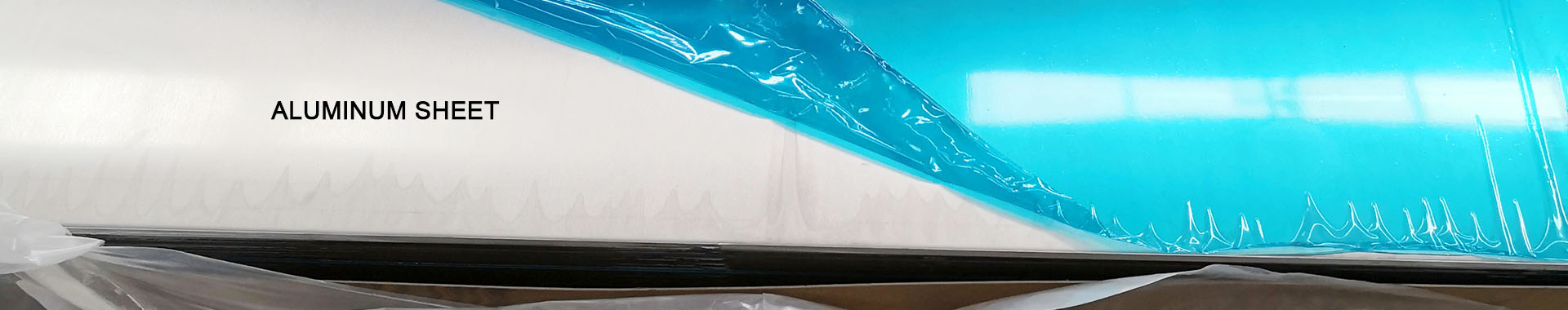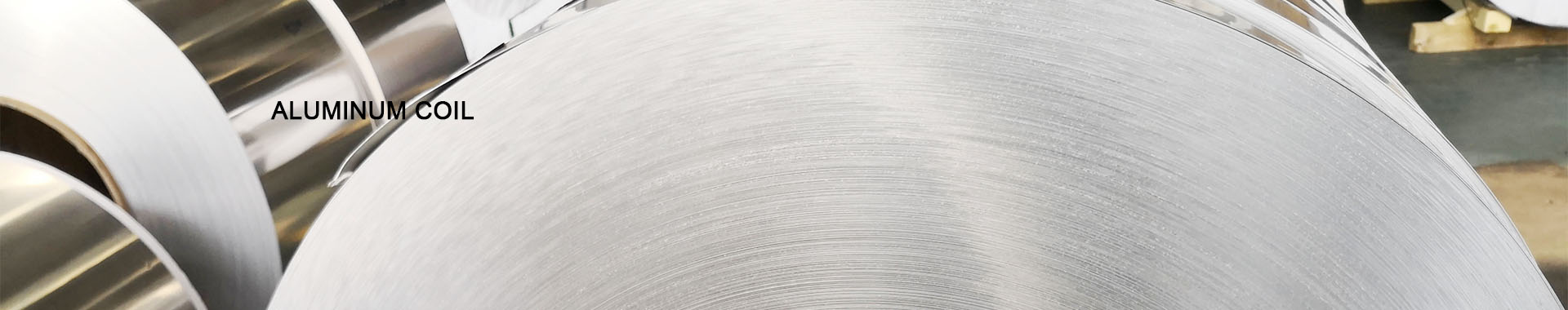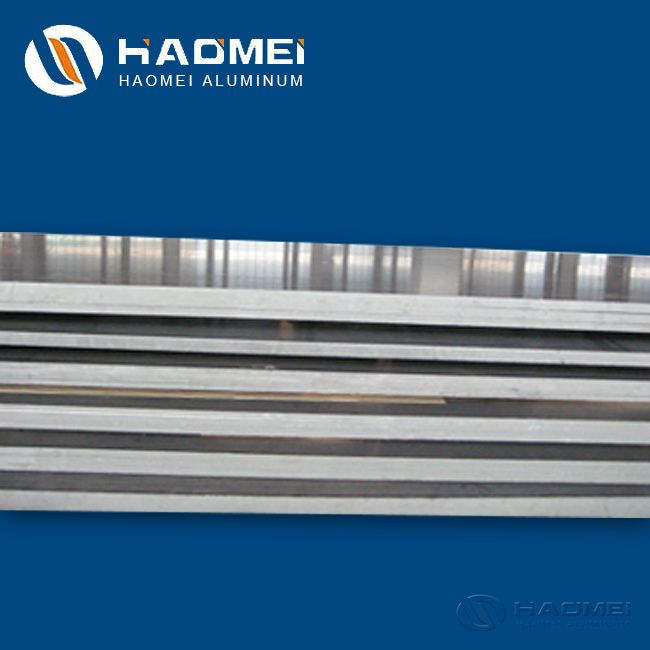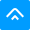Get Quot：

sale@alumhm.com
•••Home» Blog» How to Calculate 3mm Aluminium Sheet Weight

# How to Calculate 3mm Aluminium Sheet Weight

Publish: Modify: Sep 29, 2020Author: From:  HaomeiAs a big aluminium sheet supplier, Haomei Aluminum tells you how to calculate the 3mm aluminium sheet weight. Learn more.

Many customers care about how to calculate the aluminum plate weight. As an aluminium sheet supplier, Haomei Aluminum gives you a summary on the calculation method of aluminum sheet. In the formula, the thicknes is in mm, the width and length is coverted into meter. The density of aluminum plates is mostly around 2.7. For example, the density of 1060 aluminum sheet is 2.71 and that of 3003 aluminum sheet is 2.72.1. Formula for calculating the weight of aluminum sheet

Thickness (mm) * Width (m) * Length (m) * 2.71 (1060 aluminum plate density) = weight of single aluminum plate

Take the 1060 3mm aluminum sheet for example. Its width is 1.5m and length is 3m. How to calculate the 3mm aluminium sheet weight? It is 3*1.5*3*2.71=36.585 kg

2. How many pieces does 1 ton of aluminum sheets can have

Formula: 1000 kg÷single aluminum sheet weight= pieces

Take 1060 alumnum sheet for example. The specification is 3mm*1m*1m. From the first formular, we can know the single aluminum sheet weight is 8.13 kg. The number of aluminum sheets per ton is about 123.

Here are some tips about the cacluation of aluminum sheet weight:

1. Remember that the unit of thickness and the unit of length and width are different.

2. If you don’t know the density of a certain aluminum alloy, we can set it as 2.68 for 2.68 is an average densit of the aluminum sheet.

### Inquiry

 Name: Email: Tel/Whatsapp: Country: Message: SubmitHaomei Aluminum CO., LTD.

Tel/Whatsapp: +86-15978414719

Email: sale@alumhm.com

Website: https://www.alumhm.com

Xin'an Industrial Assemble Region,Luoyang,Henan Province,China